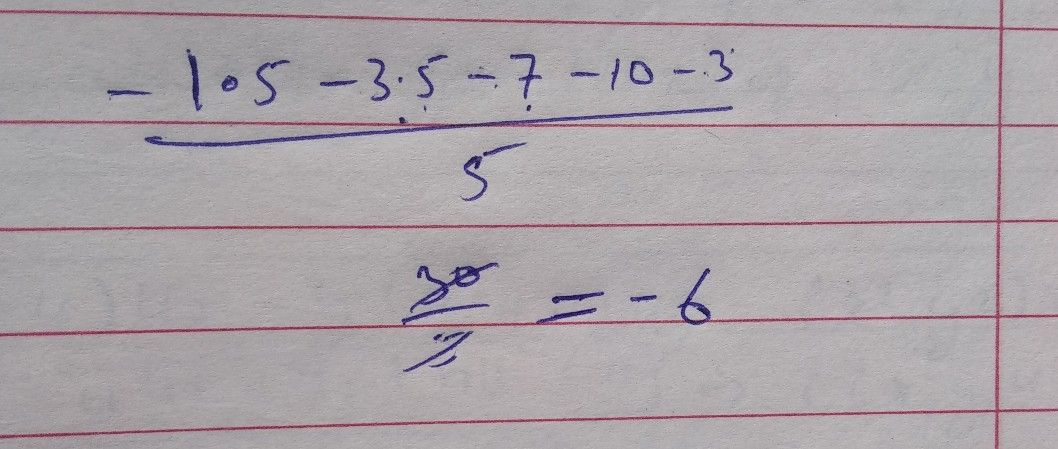Symbol
Problem$5$ $1.5$ $-3°5$ $-7$ $-10$ $-3$ Find the mean temperature.
10th-13th grade
Other
SolutionQanda teacher - PARVEEN KU